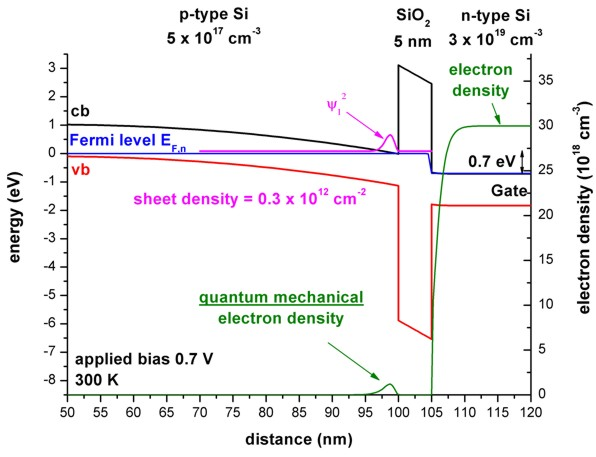nextnano.com  GUI: nextnanomat  Tool: nextnano++  Tool: nextnano³  Tool: nextnano.NEGF  Download | Search | Copyright | News | Publications  * password protected nextnano³ software1D p-Si/SiO2/poly-Si/Gate

# nextnano3 - Tutorial

## p-Si / SiO2 / poly-Si structure (MOSFET with inversion channel due to applied gate voltage)

Authors: Stefan Birner

If you want to obtain the input file that is used within this tutorial, please contact stefan.birner@nextnano.de.
`-> 1DpSi_SiO2_npolySi_Gate.in`

## p-Si / SiO2 / poly-Si structure (MOSFET with inversion channel due to applied gate voltage)

### Step 1: Layer sequence

 # width [nm] material doping 1 1 contact 2 99 p-Si 5 x 1017 cm-3 (fully ionized) 3 5 SiO2 4 54 n-Si (poly-silicon) 3 x 1019 cm-3 (fully ionized) 5 1 Gate contact

The applied gate voltage leads to confined electron states at the p-Si / SiO2 interface (n-type inversion layer) whereas the holes are repelled from the Si/SiO2 surface towards the interior of the device (i.e. to the left side).

An applied source-drain voltage in the plane of the inversion layer will lead to a flow of current which depends on the sheet density in the inversion layer.
The magnitude of the current is governed by applied gate voltage, i.e. the gate controls the sheet density and thus switches the current on or off (MOSFET, metal-oxide-semiconductor field effect transistor).### Step 2: Calculations

The temperature was set to 300 Kelvin.

Self-consistent solution of the 1D-Schrödinger-Poisson equation within single-band effective-mass approximation (using ellipsoidal effective mass tensors) for the (Delta) conduction band edges.

We vary the gate voltage from 0 V to 2.7 V in steps of 0.1 eV.

### Step 3: Results

• The following two figures show the band profiles and the electron density for two different gate voltages:
a) 0.7 V (The electron ground state is above the electron Fermi level.)
b) 2.7 V (The electron ground state is below the electron Fermi level and thus occupied, leading to a large quantum mechanical density.)In the poly-silicon on the right side of the SiO2 barrier, the electrons get depleted from the oxide interface.

Due to the fact that the Fermi level is constant outside the SiO2 barrier, no current is flowing.
(Inside the SiO2 barrier the Fermi level has a step-like feature. But as the electron density is zero inside the barrier, no current is flowing.)

The ground state electron level is associated with the longitudinal electron mass (mlongitudinal = 0.916 m0)
whereas the second and the third eigenstate (which are degenerate) are associated with the transversal electron mass (mtransversal = 0.190 m0).
Due to this degeneracy, only two rather than three Schrödinger equations have to be solved:
a) V(z), mzz = mtransversal    = 0.190 m0
b) V(z), mzz = mlongitudinal = 0.916 m0
The potential V(z) that enters into the Schrödinger equation is the same in these two cases.
``` ```The eigenvalues for mtransversal are contained in:```     ev1D_cb003_ind0**_qc001_sg001_deg001_neu_Kx001_Ky001_Kz001.dat```

The eigenvalues for mlongitudinal are contained in:```     ev1D_cb003_ind0**_qc001_sg001_deg002_neu_Kx001_Ky001_Kz001.dat```

At 2.5 eV the energy spacing between the two lowest electron states is of the order 100 meV (in the case of longitudinal effective mass).
At 2.5 eV the energy spacing between the two lowest electron states is of the order 130 meV (in the case of transversal  effective mass).
At 2.5 eV the energy spacing between the electron ground state (with longitudinal effective mass) and the ground state of the tranversal effective mass is of the order 80 meV. Thus, in this case one can safely assume that only one electron state is occupied, i.e. the electron ground state with the longitudinal mass.

• Electron sheet density in the inversion channel as a function of applied gate voltage

The file` densities/int_el_dens1D.dat `contains the integrated electron density for each region cluster.
The p-Si region, where the inversion channel is located, is termed "region cluster 2" and extends from 1 nm to 100 nm.
To plot the integrated electron charge density of the p-Si region vs. gate voltage, one has to plot the second column of this file.
To obtain the capacitance-voltage characteristics, one has to calculate the derivative of this column.• Please help us to improve our tutorial! Send comments to``` support [at] nextnano.com```.